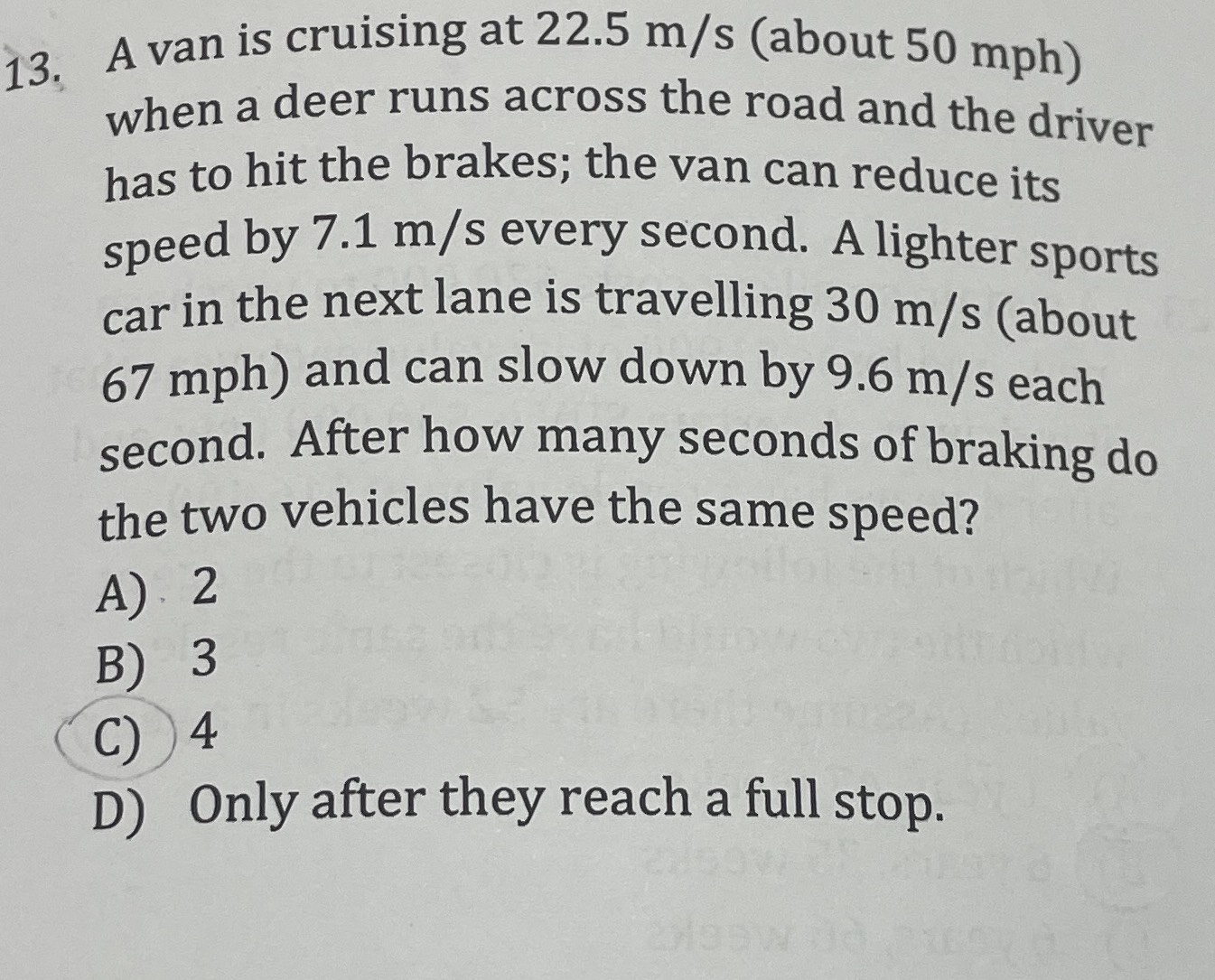### ¿Todavía tienes preguntas de matemáticas?

Pregunte a nuestros tutores expertos
Algebra
Pregunta13. A van is cruising at $$22.5 m / s$$ (about $$50 mph$$ ) when a deer runs across the road and the driver has to hit the brakes; the van can reduce its speed by $$7.1 m / s$$ every second. A lighter sports car in the next lane is travelling $$30 m / s$$ (about

$$67 mph$$ ) and can slow down by $$9.6 m / s$$ each second. After how many seconds of braking do the two vehicles have the same speed? A). $$2$$ B) $$3$$ C) $$4$$ D) Only after they reach a full stop.